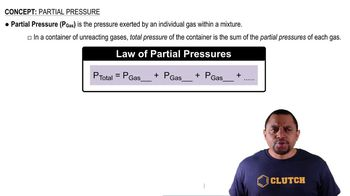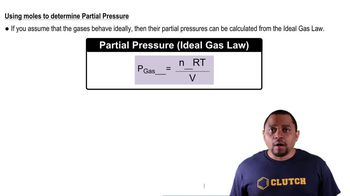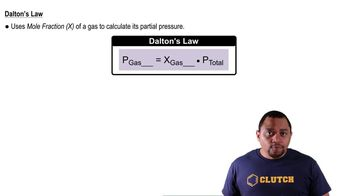Start typing, then use the up and down arrows to select an option from the list.# Partial Pressure Example 3

Jules Bruno
170views
here in this example question. It says a container has 16.7 g Oh, to 8.1 g H two and 35.2 g and to and contains a total pressure off 0.83 atmospheres were asked to complete the mole fraction about two and its partial pressure all right to determine the partial pressure vote to so pressure vote to equals the mole fraction of 02 times the pressure total. Right now we already know what the total pressure is. It is 0.83 atmospheres. So to determine partial pressure, we first have to find the mole fraction of 02 Remember, Mole fraction of O Toole equal the moles of divided by total moles of all the gasses together. So we're gonna take here. We're going to say we have 16.7 g. 02 8.1 g H two and 35.2 g and to we're going to convert each one of these grams into moles. So we look on the periodic table for the atomic masses off oxygen, hydrogen and nitrogen. Here, one mole of 02 to oxygen's comes out to 32 g. One mole of H two two hydrogen is when you add up the atomic masses is 2.16 g and then you have to nitrogen. So one mole event two is 28 02 g. Here, all of our grants cancel out and we'll have the moles for each one of these gasses. Or each one of these. Yeah, each one of these gasses. So that's gonna come out 2.5 to 19 moles of 02 4.179 moles H two and 1. Moles of end to So take those and plug them in. So we have 5 to 19 moles of on the bottom. We have the collective moles of everyone. So here we're just adding them all together. Okay, And then when you work that out, you'll get your mole fraction for 02 which comes out to be 20.900 Take that mole fraction and plug it in here. So here's our mole. Fraction of vote to remember. Mole Fraction is a unit list number, so then 0.900 times 0.83 atmospheres comes out 2. atmospheres here are answer has two significant figures because our lowest number of sick figs are 8.1 and 0. they both have to sick fix. So just remember, utilizing Dalton's law, we can use the mole fraction of any gas times the pressure total to find the partial pressure of that particular gas.00:4402:0900:4803:2600:2703:18